# Resources tagged with: Visualising

Filter by: Content type:
Age range:
Challenge level:

There are 179 NRICH Mathematical resources connected to Visualising, you may find related items under Thinking Mathematically.

Broad Topics > Thinking Mathematically > Visualising### Polygon Pictures

##### Age 11 to 14Challenge Level

Can you work out how these polygon pictures were drawn, and use that to figure out their angles?### Polygon Rings

##### Age 11 to 14Challenge Level

Join pentagons together edge to edge. Will they form a ring?### Seven Squares

##### Age 11 to 14Challenge Level

Watch these videos to see how Phoebe, Alice and Luke chose to draw 7 squares. How would they draw 100?### Partly Painted Cube

##### Age 14 to 16Challenge Level

Jo made a cube from some smaller cubes, painted some of the faces of the large cube, and then took it apart again. 45 small cubes had no paint on them at all. How many small cubes did Jo use?### Mystic Rose

##### Age 14 to 16Challenge Level

Use the animation to help you work out how many lines are needed to draw mystic roses of different sizes.### Diminishing Returns

##### Age 11 to 14Challenge Level

How much of the square is coloured blue? How will the pattern continue?### Tower of Hanoi

##### Age 11 to 14Challenge Level

The Tower of Hanoi is an ancient mathematical challenge. Working on the building blocks may help you to explain the patterns you notice.### Christmas Chocolates

##### Age 11 to 14Challenge Level

How could Penny, Tom and Matthew work out how many chocolates there are in different sized boxes?### Baravelle

##### Age 7 to 16Challenge Level

What can you see? What do you notice? What questions can you ask?### Cuboid Challenge

##### Age 11 to 16Challenge Level

What's the largest volume of box you can make from a square of paper?### Eight Hidden Squares

##### Age 7 to 14Challenge Level

On the graph there are 28 marked points. These points all mark the vertices (corners) of eight hidden squares. Can you find the eight hidden squares?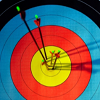### Spotting the Loophole

##### Age 14 to 16Challenge Level

A visualisation problem in which you search for vectors which sum to zero from a jumble of arrows. Will your eyes be quicker than algebra?### Route to Infinity

##### Age 11 to 14Challenge Level

Can you describe this route to infinity? Where will the arrows take you next?### Squares in Rectangles

##### Age 11 to 14Challenge Level

A 2 by 3 rectangle contains 8 squares and a 3 by 4 rectangle contains 20 squares. What size rectangle(s) contain(s) exactly 100 squares? Can you find them all?### Semi-regular Tessellations

##### Age 11 to 16Challenge Level

Semi-regular tessellations combine two or more different regular polygons to fill the plane. Can you find all the semi-regular tessellations?### Cubes Within Cubes Revisited

##### Age 11 to 14Challenge Level

Imagine starting with one yellow cube and covering it all over with a single layer of red cubes, and then covering that cube with a layer of blue cubes. How many red and blue cubes would you need?### Square Coordinates

##### Age 11 to 14Challenge Level

A tilted square is a square with no horizontal sides. Can you devise a general instruction for the construction of a square when you are given just one of its sides?### Isosceles Triangles

##### Age 11 to 14Challenge Level

Draw some isosceles triangles with an area of $9$cm$^2$ and a vertex at (20,20). If all the vertices must have whole number coordinates, how many is it possible to draw?### Efficient Cutting

##### Age 11 to 14Challenge Level

Use a single sheet of A4 paper and make a cylinder having the greatest possible volume. The cylinder must be closed off by a circle at each end.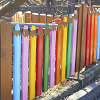### Fence It

##### Age 11 to 14Challenge Level

If you have only 40 metres of fencing available, what is the maximum area of land you can fence off?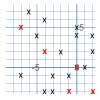### Ten Hidden Squares

##### Age 7 to 14Challenge Level

These points all mark the vertices (corners) of ten hidden squares. Can you find the 10 hidden squares?### Square It

##### Age 11 to 16Challenge Level

Players take it in turns to choose a dot on the grid. The winner is the first to have four dots that can be joined to form a square.### On the Edge

##### Age 11 to 14Challenge Level

If you move the tiles around, can you make squares with different coloured edges?### Cuboids

##### Age 11 to 14Challenge Level

Can you find a cuboid that has a surface area of exactly 100 square units. Is there more than one? Can you find them all?### The Spider and the Fly

##### Age 14 to 16Challenge Level

A spider is sitting in the middle of one of the smallest walls in a room and a fly is resting beside the window. What is the shortest distance the spider would have to crawl to catch the fly?### Painted Cube

##### Age 14 to 16Challenge Level

Imagine a large cube made from small red cubes being dropped into a pot of yellow paint. How many of the small cubes will have yellow paint on their faces?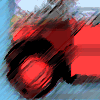### Buses

##### Age 11 to 14Challenge Level

A bus route has a total duration of 40 minutes. Every 10 minutes, two buses set out, one from each end. How many buses will one bus meet on its way from one end to the other end?### Coordinate Patterns

##### Age 11 to 14Challenge Level

Charlie and Alison have been drawing patterns on coordinate grids. Can you picture where the patterns lead?### Seven Squares - Group-worthy Task

##### Age 11 to 14Challenge Level

Choose a couple of the sequences. Try to picture how to make the next, and the next, and the next... Can you describe your reasoning?### Picturing Square Numbers

##### Age 11 to 14Challenge Level

Square numbers can be represented as the sum of consecutive odd numbers. What is the sum of 1 + 3 + ..... + 149 + 151 + 153?### Picturing Triangular Numbers

##### Age 11 to 14Challenge Level

Triangular numbers can be represented by a triangular array of squares. What do you notice about the sum of identical triangle numbers?### An Unusual Shape

##### Age 11 to 14Challenge Level

Can you maximise the area available to a grazing goat?### Rolling Around

##### Age 11 to 14Challenge Level

A circle rolls around the outside edge of a square so that its circumference always touches the edge of the square. Can you describe the locus of the centre of the circle?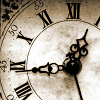### John's Train Is on Time

##### Age 11 to 14Challenge Level

A train leaves on time. After it has gone 8 miles (at 33mph) the driver looks at his watch and sees that the hour hand is exactly over the minute hand. When did the train leave the station?##### Age 11 to 14Challenge Level

How many different symmetrical shapes can you make by shading triangles or squares?### Reflecting Squarely

##### Age 11 to 14Challenge Level

In how many ways can you fit all three pieces together to make shapes with line symmetry?### Proofs with Pictures

##### Age 14 to 18

Some diagrammatic 'proofs' of algebraic identities and inequalities.### Triangles to Tetrahedra

##### Age 11 to 14Challenge Level

Imagine you have an unlimited number of four types of triangle. How many different tetrahedra can you make?### Cubes Within Cubes

##### Age 7 to 14Challenge Level

We start with one yellow cube and build around it to make a 3x3x3 cube with red cubes. Then we build around that red cube with blue cubes and so on. How many cubes of each colour have we used?### Marbles in a Box

##### Age 11 to 16Challenge Level

How many winning lines can you make in a three-dimensional version of noughts and crosses?### Nine Colours

##### Age 11 to 16Challenge Level

Can you use small coloured cubes to make a 3 by 3 by 3 cube so that each face of the bigger cube contains one of each colour?### One and Three

##### Age 14 to 16Challenge Level

Two motorboats travelling up and down a lake at constant speeds leave opposite ends A and B at the same instant, passing each other, for the first time 600 metres from A, and on their return, 400. . . .##### Age 14 to 16Challenge Level

In this problem we are faced with an apparently easy area problem, but it has gone horribly wrong! What happened?### Is There a Theorem?

##### Age 11 to 14Challenge Level

Draw a square. A second square of the same size slides around the first always maintaining contact and keeping the same orientation. How far does the dot travel?### Three Cubes

##### Age 14 to 16Challenge Level

Can you work out the dimensions of the three cubes?### Picture Story

##### Age 14 to 16Challenge Level

Can you see how this picture illustrates the formula for the sum of the first six cube numbers?##### Age 14 to 16Challenge Level

Four rods are hinged at their ends to form a convex quadrilateral. Investigate the different shapes that the quadrilateral can take. Be patient this problem may be slow to load.### Vanishing Point

##### Age 14 to 18Challenge Level

How can visual patterns be used to prove sums of series?### The Farmers' Field Boundary

##### Age 11 to 14Challenge Level

The farmers want to redraw their field boundary but keep the area the same. Can you advise them?### Constructing Triangles

##### Age 11 to 14Challenge Level

Generate three random numbers to determine the side lengths of a triangle. What triangles can you draw?